# Date & Time in tkinter Window

Basics of Python Tkinter More Projects in Tkinter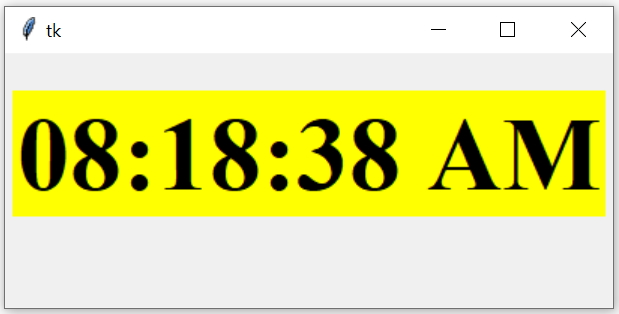Tkinter GUI displaying real time clock with Weekday and date in different formats
``````import tkinter  as tk
from tkinter import ttk
my_w = tk.Tk()
my_w.geometry("405x170")
from time import strftime
def my_time():
time_string = strftime('%H:%M:%S %p') # time format
l1.config(text=time_string)
l1.after(1000,my_time) # time delay of 1000 milliseconds

my_font=('times',52,'bold') # display size and style

l1=tk.Label(my_w,font=my_font,bg='yellow')

my_time()
my_w.mainloop()``````
We are calling the same function my_time() at an interval of 1 sec ( 1000 milliseconds ) by using `l1.after(1000,my_time)`

## Managing Clock display format

Add date and Month name in next line. Here we changed the background colour of the Label to `bg='#53fcf7'`
``time_string = strftime('%H:%M:%S %p \n %d %B')``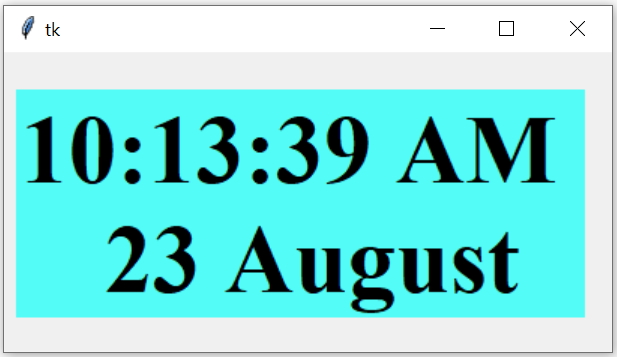Display Local date ( %x ) along with clock.
``time_string = strftime('%H:%M:%S %p \n %x')``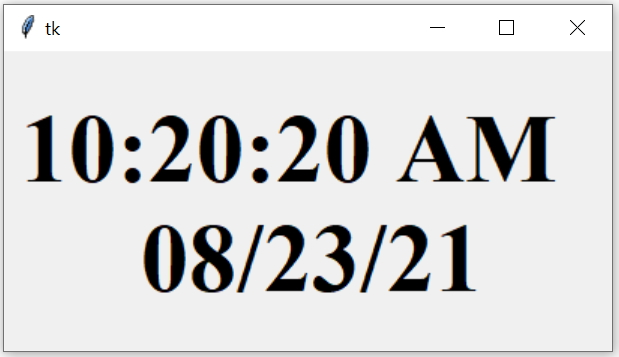With Full weekday
``time_string = strftime('%H:%M:%S %p \n %A \n %x')``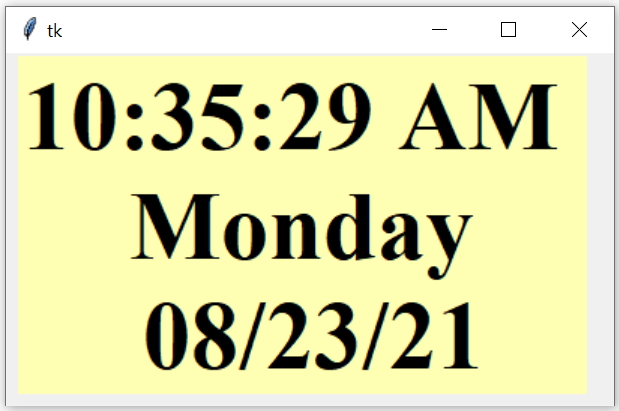Here is a list of formats can be used with strftime()

## Adding time to Entry box on Button click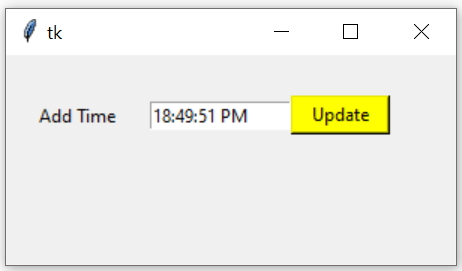``````import tkinter  as tk
from tkinter import ttk
my_w = tk.Tk()
my_w.geometry("300x140")
from time import strftime
def my_time(): # On button click
time_string = strftime('%H:%M:%S %p')
e1_str.set(time_string)  # adding time to Entry

l1 = tk.Label(my_w,  text='Add Time', width=10 )
e1_str=tk.StringVar()
e1 = tk.Entry(my_w, textvariable=e1_str,width=15) #  Entry box
e1.grid(row=1,column=2)

b1 = tk.Button(my_w, text='Update', width=8,bg='yellow',
command=lambda: my_time())
b1.grid(row=1,column=3)

my_w.mainloop()``````
One more set of input and buttons can be added to record the start time and end time of any process.

## List of formats used for generating date and time

FormatMeaning
%aLocale’s abbreviated weekday name.
%ALocale’s full weekday name.
%bLocale’s abbreviated month name.
%BLocale’s full month name.
%cLocale’s appropriate date and time representation.
%dDay of the month as a decimal number [01,31].
%HHour (24-hour clock) as a decimal number [00,23].
%IHour (12-hour clock) as a decimal number [01,12].
%jDay of the year as a decimal number [001,366].
%mMonth as a decimal number[01,12].
%MMinute as a decimal number[00,59].
%pLocale’s equivalent of either AM or PM.
%SSecond as a decimal number[00,61].
%UWeek number of the year(Sunday as the first day of the week) as a decimal number[00,53]. All days in a new year preceding the first Sunday are considered to be in week 0.
%wWeekday as a decimal number [0(Sunday),6].
%WWeek number of the year (Monday as the first day of the week) as a decimal number[00,53]. All days in a new year preceding the first Monday are considered to be in week 0.
%x Locale’s appropriate date representation.
%XLocale’s appropriate time representation.
%yYear without century as a decimal number [00,99].
%YYear with century as a decimal number.
%ZTime zone name (no characters if no time zone exists).
%%A literal '%' character.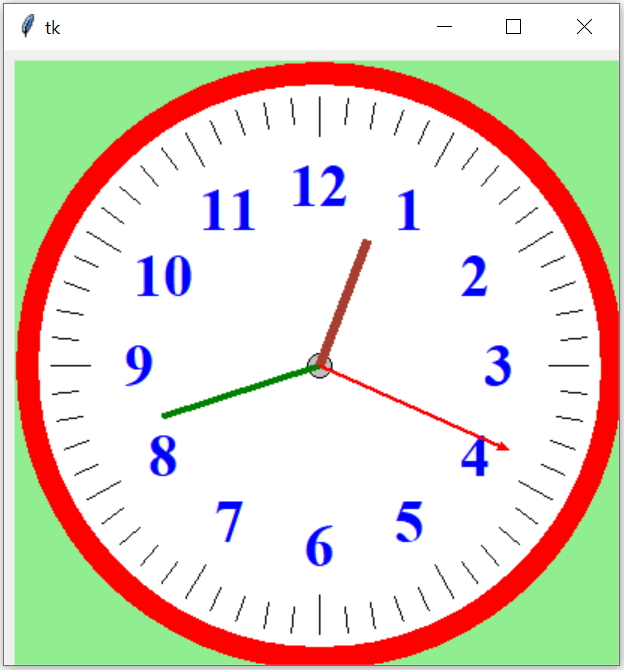Subscribe to our YouTube Channel here

## Subscribe

* indicates required
Subscribe to plus2netplus2net.com

 20-02-2023 Thanks a lot